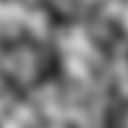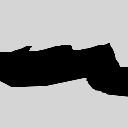# teaching machines

## CS 455 Lecture 17 – Heightmaps

April 9, 2015 by . Filed under cs455, lectures, spring 2015.

### Agenda

• what ?s
• heightmap exercise

### TODO

Heightmaps are grayscale images used to generate terrain. For example, to get a landscape with rolling hills, we can use a noisy but smooth image:A noise image used to generate a hilly terrain.

To produce a plateau (white) with a sudden chasm (black), we can use this image of starker contrast:A heightmap for a plateau with a dramatic rift between surfaces.

The are two significant advantages of a heightmap over a traditional 3D mesh:

1. They can be generated and edited in many different tools.
2. They are backed by a very simple and fast data structure: an array.

The one drawback to heightmaps is that they are not expressed as vertices and triangles. To render our terrain, we’ll first need to convert the heightmap into suitable geometry that we can ship to the GPU. Your task in lab, then, is to write class Heightmap class that reads in an image and produces vertex attributes (position and normal) and connectivity information (the indices of the vertices forming each face).

1. In the Heightmap constructor, read the Image at the specified path. Let the image’s width be the terrain’s width. Let the image’s height be the terrain’s depth. Let the grayscale intensity at index (x, z) be used as the height of the terrain at location (x, z). However, consider the grayscale value (which is in [0, 255]) as a proportion of the max parameter.
2. Write a destructor.
3. Write GetWidth and GetDepth.
4. Write operator()(int x, int z). Return as a reference the height at location (x, z) in the terrain.
5. Complete ToAttributes. I suggest tackling it in the following steps:
1. Determine the number of vertices.
2. Generate the list of vertex positions in the terrain. Each pixel in the original image corresponds to a vertex. What is each pixel’s location? Its location in the image determines its xz-coordinates, and its grayscale intensity determines its y-coordinate.
3. Prime the list of vertex normals to initially all have 0 length.
4. Determine the number of faces.
5. Generate the list of vertex indices that connect up to form the terrain’s triangles. When you have identified a face’s vertices, calculate its normal as the crossproduct of two of its sides. Don’t forget to normalize. Add this face normal to each of its three vertices’ normals. ObjUtilities contains code that might serve as a template.
6. Renormalize all vertex normals.
6. Test your solution in the heightmap project, which uses your Heightmap to load models/noise.pgm.

Complete this lab exercise and send a screenshot.

Next week, we’ll look at automatically adjusting the camera’s height based on its position in the terrain.

### Haiku

a small step for mankind
Mankind has evolved
We once thought the world was flat